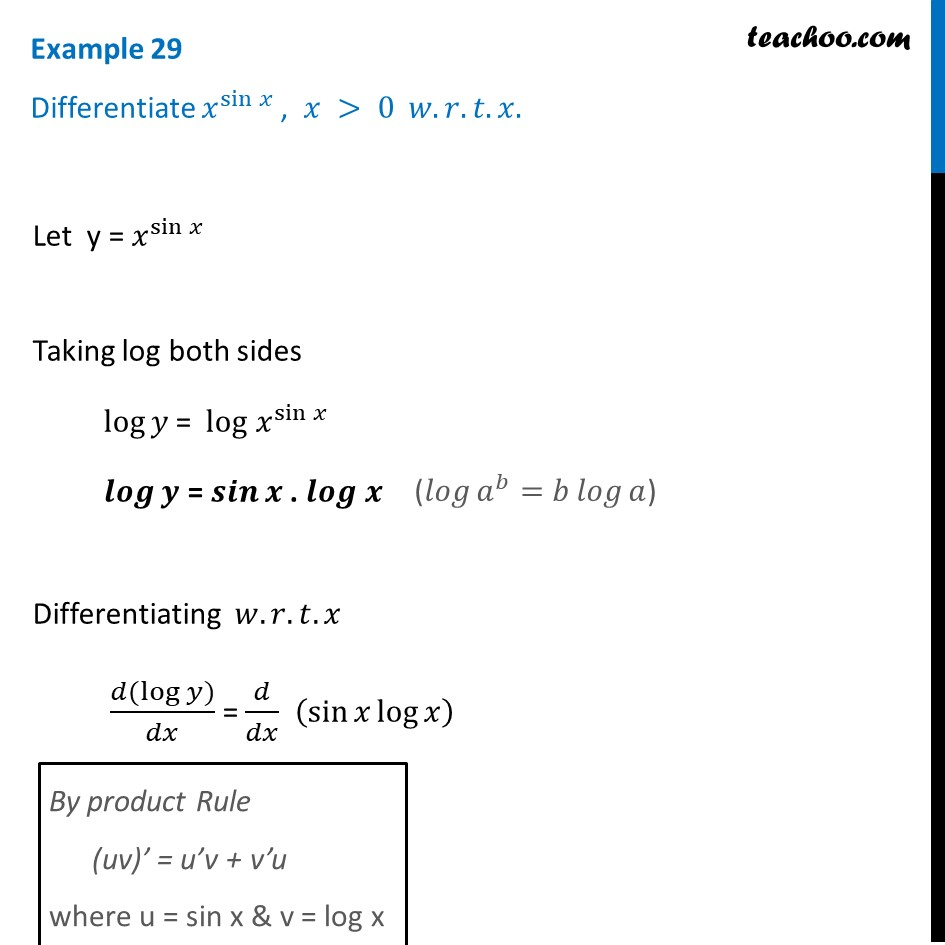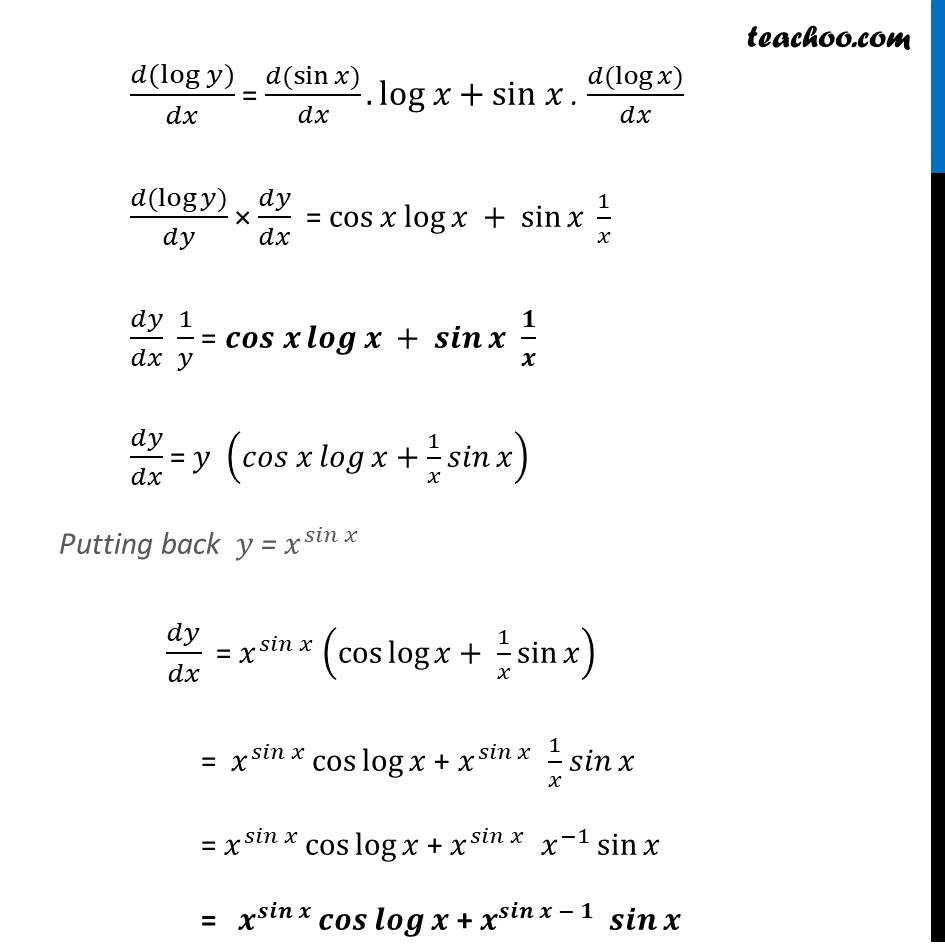Chapter 5 Class 12 Continuity and Differentiability

Class 12
Important Questions for exams Class 12Learn in your speed, with individual attention - Teachoo Maths 1-on-1 Class

### Transcript

Example 29 Differentiate 𝑥^sin⁡𝑥 , 𝑥 > 0 𝑤.𝑟.𝑡. 𝑥.Let y = 𝑥^sin⁡𝑥 Taking log both sides log⁡𝑦 = log 𝑥^sin⁡𝑥 𝒍𝒐𝒈⁡𝒚 = 𝒔𝒊𝒏⁡𝒙 . 𝒍𝒐𝒈 𝒙 Differentiating 𝑤.𝑟.𝑡.𝑥 (𝑑(log⁡〖𝑦)〗)/𝑑𝑥 = 𝑑/𝑑𝑥 (sin⁡〖𝑥 log⁡𝑥 〗 ) By product Rule (uv)’ = u’v + v’u where u = sin x & v = log x (𝑑(log⁡〖𝑦)〗)/𝑑𝑥 = (𝑑(sin⁡𝑥))/𝑑𝑥.log 𝑥+sin 𝑥 . (𝑑(log⁡𝑥))/𝑑𝑥 (𝑑(log⁡〖𝑦)〗)/𝑑𝑦 × 𝑑𝑦/𝑑𝑥 = cos⁡𝑥 log⁡𝑥 + sin⁡𝑥 1/𝑥 𝑑𝑦/𝑑𝑥 1/𝑦 = 𝒄𝒐𝒔 𝒙⁡𝒍𝒐𝒈⁡𝒙 + 𝒔𝒊𝒏⁡𝒙 𝟏/𝒙 𝑑𝑦/𝑑𝑥 = 𝑦 (〖𝑐𝑜𝑠 𝑥〗⁡〖𝑙𝑜𝑔⁡〖𝑥+1/𝑥〗 𝑠𝑖𝑛⁡𝑥 〗 ) Putting back 𝑦 = 𝑥^𝑠𝑖𝑛⁡𝑥 𝑑𝑦/𝑑𝑥 = 𝑥^𝑠𝑖𝑛⁡𝑥 (cos⁡〖log⁡〖𝑥+ 1/𝑥〗 sin⁡𝑥 〗 ) = 𝑥^𝑠𝑖𝑛⁡𝑥 cos⁡log⁡𝑥 + 𝑥^𝑠𝑖𝑛⁡𝑥 1/𝑥 𝑠𝑖𝑛⁡𝑥 = 𝑥^𝑠𝑖𝑛⁡𝑥 cos⁡log⁡𝑥 + 𝑥^𝑠𝑖𝑛⁡𝑥 𝑥^(−1) sin⁡𝑥 = 𝒙^𝒔𝒊𝒏⁡𝒙 𝒄𝒐𝒔⁡𝒍𝒐𝒈⁡𝒙 + 𝒙^𝒔𝒊𝒏⁡〖𝒙 − 𝟏〗 𝒔𝒊𝒏⁡𝒙